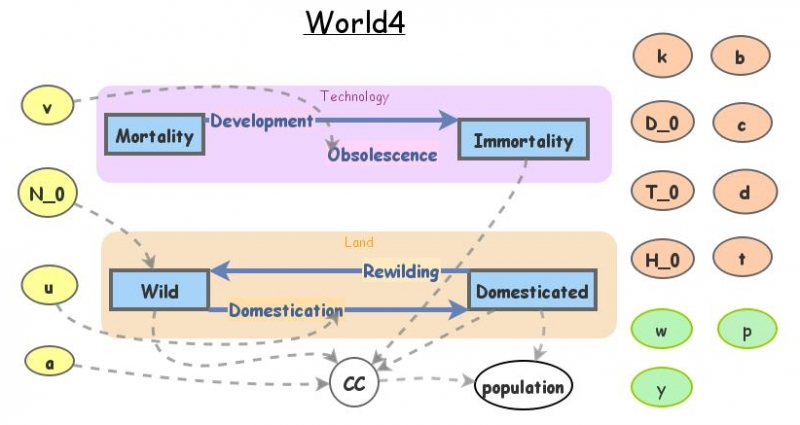World4.5World 4 is a predictive model for world population. Population has grown hyper-exponentially in the last millenium, with the doubling time decreasing from 900 years  in 1000 CE to a minimum of ~35 years in 1963 CE. Technology is defined as that which decreases the death rate and/or increases the effective birth rate (i.e. by decreasing infant mortality). Technology grows exponentially, therefore population fits a hyper-exponential (exponent within an exponent). Models for the end of growth are explored using equations that express the ways humans are depleting Earth's biocapacity, the nature of resource depletion, and the relationship between natural resources and human carrying capacity. This simple model, containing just two closed systems, captures the subtle shifts in the population trajectory of the last 50 years. Specifically, hyperexponential growth has given way to subexponential growth. A peak is predicted for the time around 2030.